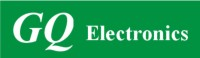Active Users: / Visits Today:
Highest Active Users:
GQ Electronics Technical Support Forum
Home | Profile | Register | Active Topics | Members | Search | FAQAll Forums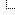GQ Electronics Forums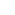3. GQ EMF EF Meter RF Spectrum Power Analyzer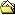RF Alarm at 1 Microwatt/cmý and -40dbm ???

Note: You must be registered in order to post a reply.

Screensize:
Format Mode: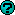Format: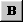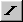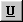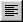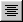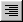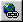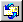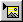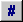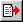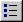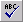Message:

* HTML is OFF
* Forum Code is ON
 Smilies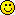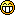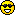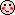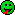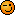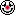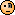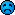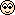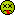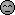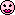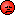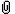Insert an Image File
Check here to include your profile signature.

 T O P I C    R E V I E W frank.mathieu@GQ Posted - 08/22/2021 : 06:13:05 If this question has been answered already, then I am sorry. I did not find the answer.I want to set the alarm of my EMF-390 to 100 Nano Watts per cmý.The RF Alarm currently says 1.0 Micro Watts per cmý -40dbm The alarm is beeping at 1 Micro Watts which I understand and I am happy with.What confuses me is -40dbm.I thought the formula for dbm is based on mW per square meter - not centimeterý.I assume that1 Micro-watt = 1/1000000 Watts 1 Milli-watt = 1/1000 Watts1 Micro-watt = 1/1000 Milli-Watts1 square meter is 100cm by 100cm = 10000 cmýTo my knowledge, the dbm formula should be based on Milli-Watts per mý.1 Micro-watt per cmý is 1/1000 Milliwatts per cmý1/1000 mW/cmý * 10000cmý/mý is 10mW/mýlog10(10mW/mý)*10 = 10dbmAm I correct?I have a feeling that the RF-Alarm screen in my GQ EMF-390v2Re3.69 may not convert cmý to mý.Maybe, I miss something, Please let me know.As for now, I have set the RF Alarm to -50dbm, which is converted to 100nW/cmý by the RF-Alarm settings. The alarm works as expected.I just want to have a confirmation that 100nW/cmý really is 0dbm, not -50dbm.Thank you, Frank. 20   L A T E S T    R E P L I E S    (Newest First) Damien68 Posted - 09/10/2021 : 22:23:01 EMF-390 should show ''-30dBm'' for a power density of 10uW/cm2.this is only an order of magnitude because EMF-390 will not replace a laboratory device calibrated specifically on the frequencies that you want to examine. but it is a good representation. karpunoleg Posted - 09/10/2021 : 11:47:56 So what exactly should the device show at 10 MkW / square centimeter? In Russia, is this the maximum permissible value of the power flux density? Is it possible to give a photo of such a measurement? In order not to make measurement errors. EmfDev Posted - 08/30/2021 : 09:48:01 That's great! Thank you also for your post, and thank you @Damien68 for helping. Damien68 Posted - 08/28/2021 : 05:24:24 your summary is exact, thank to you for this post Frank.I also think it could be useful to other people. frank.mathieu@GQ Posted - 08/28/2021 : 04:33:19 Please let me summarize the topic for people reading it.I originally asked how a power density of 1 Microwatt per cmý can be -40 dBm.By the time I assumed that power densities are described in mW/mý and dBm, which is not correct. The unit dBm is only valid for power, not power density.Reply #11 explained that for a power density of 10mW/cm2, the EMF-390 measures 1mW (0 dBm) power. As a result, 1mW/cmý is measured with 1/10th mW = 10^(-1)mW or -10dBm (reply #6). Mathematically, 1 microWatt/cmý is 1/1000th milliWatts/cmý = 1*10^(-3)mW/cmý. When one considers the above conversion coefficient of 1/10, one microWatt/cmý is received as 1/10000mW.In other words, EMF-390 measures a power of 1/10000mW (-40dbm) when a power density of 1microW/cmý is at it's antenna.In short, dBm can be used to depict power, NOT power densities. The only unit for power density is power over an area (for example mW/mý or mW/cmý or microWatt/cmý).Power is dependent on the signal strengths coming from the sending antenna(s), on directions, and on the parameters of the receiving antenna (which are described above).The RF Alarm screen depicts "power" measured by the device in dBm and the associated "power density" in "power / area" that is calculated via the conversion logic.Let me thank EmfDev and Damien68 again. frank.mathieu@GQ Posted - 08/27/2021 : 03:04:19 Understood,Thank you both. EmfDev Posted - 08/26/2021 : 11:16:14 Another way for looking at it.The field mW/m2 depends on the transmitter or source. For example, if there is only one RF Transmitter/Tower 10meters away, then in your location there is going to be an RF field or RF Power Density. Let say, for this example, the EXACT field in your location is 10 mW/cm2. (this field or power density is based on the transmitter strength).Now, if you place an antenna on the same location 10meters away, the antenna can detect/receive the signal/field and this is usually expressed in Power(e.g. mW) or dBm. A good antenna can detect better which translates to higher Power or dBm compared to a poor antenna which would measure lower Power or dBm. For example a good antenna can detect 0dBm, while a poor another can detect -50dBm.The 2 different antennas measure different Power but they must convert to the same RF Power Density of 10mW/cm2 or close to it for better accuracy. Here is where the other factors come in when calculating the correct power density. Damien68 Posted - 08/26/2021 : 10:49:18 quote:Originally posted by frank.mathieu@GQI agree honestly, my questions are awkward, absurd, without physical meaning and without sense - I am in no way skilled in this area which is why I do not use the terminology precisely enough. For that I hope you accept my apology. Still, I am trying to get a grasp on what you wrote:'when the power density of field is: 10mW/cm2, the EMF-390 receive on is antenna 1mW (0 dBm), we can determine with these data the conversion coefficient between the power received by the EMF-390 antenna (dBm) and the power density of the field (mW/cm2).'I think I understand it, but please let me take the apart the sentence to make sure I really do.a) 1mW is a power value measured within EMF-390, correct?b) 0 dBm is the result of the equation log10(1mW)*10, correct?c) 10mW/cmý is a result of measured values and the conversion coefficient, correct?That leads me to my final question:d) does a mathematical/physical equivalent dBm or dBm/mý for the power density 10mW/cmý exist at all?Thanks a lot.yes there is no problem, if I point out that your question is awkward it is by no means a complaint, but just to indicate that you are trying to understand something that is not consistent. It also happens to me from time to time, so you have to reconsider your prerequisites.a, b, c is OKd: we cannot express a power density in dBm/cm2 because the dBm scale is no longer linear but logarithmic and the ratio (power/area) is not constant if power scale is not linear.demonstration:if we have a homogeneous field of 10mW/cm2.to calculate the power of this field on a surface of 2 cm2 we obtain:2 cm2 * 10mW/cm2 = 20mW.if we try to convert 10mW/cm2 to dBm/cm2:10mW = 10dBmso: 10mW/cm2 would be equivalent to: 10dBm/cm2if we calculate the power of this field on the same surface of 2 cm2 we would obtain:2 cm2 * 10dBm/cm2 = 20dBm = 100 mW which is false since the previous calculation gives us 20mW. The error comes from the fact that in this case the power/area ratio is no longer constant for the reason stated above.therefore we cannot express a power density in dBm/cm2. frank.mathieu@GQ Posted - 08/26/2021 : 10:04:04 I agree honestly, my questions are awkward, absurd, without physical meaning and without sense - I am in no way skilled in this area which is why I do not use the terminology precisely enough. For that I hope you accept my apology. Still, I am trying to get a grasp on what you wrote:'when the power density of field is: 10mW/cm2, the EMF-390 receive on is antenna 1mW (0 dBm), we can determine with these data the conversion coefficient between the power received by the EMF-390 antenna (dBm) and the power density of the field (mW/cm2).'I think I understand it, but please let me take apart the sentence to make sure I really do.a) 1mW is a power value measured within EMF-390, correct?b) 0 dBm is the result of the equation log10(1mW)*10, correct?c) 10mW/cmý is a result of measured values and the conversion coefficient, correct?That leads me to my final question:d) does a mathematical/physical equivalent dBm or dBm/mý for the power density 10mW/cmý exist at all?Thanks a lot. Damien68 Posted - 08/26/2021 : 00:37:46 quote:Originally posted by frank.mathieu@GQIn other words, my question is: When the device shows a power density of 1mW/mý, what would be the power of the (electromagnetic?) field around the device in mW and dBm?Thank you.hi Frank,your question is very awkward and makes no sense.the power of a field is always expressed by its power density (mW/cm2). never by direct power (mW or dBm)when we speak of the power of a field in mW or dBm, we always speak of the power received by a device (by its antenna).depending on the device, for the same field, depending on the size of its antenna, we can have a more or less strong received power, therefore more or less high dBm. this cannot therefore strictly characterize the power of a field, but gives relative information which with a calibration coefficient (which depends on the device) can be converted into power density.when the power density of field is: 10mW/cm2, the EMF-390 receive on is antenna 1mW (0 dBm), we can determine with these data the convertion coefficient between the power received by the EMF-390 antenna (dBm) and the power density of the field (mW/cm2).wanting to express the power of a field by a direct power has no physical meaning, it is absurd.with a direct power you can only express the globally power transmitted or received with for example a smartphone, a wifi device, a RF meter, ... . EmfDev Posted - 08/25/2021 : 14:14:35 quote:Originally posted by frank.mathieu@GQIn other words, my question is: When the device shows a power density of 1mW/mý, what would be the power of the (electromagnetic?) field around the device in mW and dBm?Thank you.1mW/m2 should correspond to -50dBm = 0.00001 mW EmfDev Posted - 08/25/2021 : 14:10:35 quote:Originally posted by frank.mathieu@GQThe formula that I was told to use for converting mW/m2 to dBm is log10(x mW/m2)*10. This formula I have mentioned earlier already. You confirmed that this is correct.I probably missed this and can now confirm that this formula is wrong. dBm is log10(PmW)*10.quote:Originally posted by frank.mathieu@GQWhat formula is used to receive 100000mW for 0dbm as you said in your last comment?should be 100000mW/m20 dBm = 1mW then using power density formula, = 100000 mW/m2 frank.mathieu@GQ Posted - 08/25/2021 : 07:58:31 In other words, my question is: When the device shows a power density of 1mW/mý, what would be the power of the (electromagnetic?) field around the device in mW and dBm?Thank you. frank.mathieu@GQ Posted - 08/25/2021 : 05:38:02 Good morning, I agree that the formula in your first sentence correctly defines the Power density which is power divided by area.It's result could be any value - very small and very large. I agree also with you that Power density is Power over an Area, which is usually expressed in this case mW/m2.The formula that I was told to use for converting mW/mý to dBm is log10(x mW/mý)*10. This formula I have mentioned earlier already. You confirmed that this is correct.1mW on a surface of 1m by 1m make 1mW/mý. When I enter this value into the formula above, the result is:log10(1)*10 = 0.00 dBm.As I said earlier, the reverse operation is mW/mý = 10^(dBm Value / 10)Now, lets take your example a 1meter x 1meter surface, a 0dBm.I enter this value 0 into the formula 10^(0/10). The result is 1mW/mý.What formula is used to receive 100000mW for 0dbm as you said in your last comment?Thanks again, Frank. EmfDev Posted - 08/24/2021 : 13:40:47 The Power in mW or dBm can be compared to cell phone signal strength. The cell phone receives that much RF power/signal in a specific location. Power density can then be calculated using the formula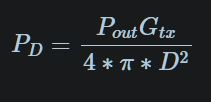.In your example/question, -10dBm is not a power density but is same as the signal strength. It is the magnitude of the signal received.Power density is Power over an Area, which is usually expressed in this case mW/m2. In a 1meter x 1meter surface, a 0dBm RF signal would result 100000mW in power. frank.mathieu@GQ Posted - 08/24/2021 : 07:07:14 I hope I understand you correctly.We are talking about two values or two scales:1. the power that an electromagnetic field has and 2. the power density that is measured by the device.The device converts these values based on Density gain, Antenna dimensions, calibration, etc.As a result, a) an electromagnetic field with a power of 0dbm or 1mW will be received by the device with a density of 10mW/cmý or 100000mW/mý.b) an electromagnetic field with a power of -40dbm or 0.0001mW will be received with a density of 0.001mW/cmý or 10mW/mý. This 'measured' power density of 0.001 mW/cmý is the same as 1uW/cmý (1uW/cmý = 1000*mW/cmý). c) mathematically speaking, -40dbm represent 0.0001mW/mýd) It seems, I was wrong because I thought the values I mentioned in (c) were displayed in the RF-Alarm screen and they are correct.e) the RF-Alarm screen does shows the correlation between power density that is 'measured' by EMF-390 (1uW/cmý) and the power of the electric field that according to calibration and gain settings would be necessary to generate this reading: -40dbm. If all these assumptions are correct, then I am not sure if my formula for converting pW/cmý into dbm is applicableWhen the device warned me of values starting at 1uW/cmý, I thought that would represent an electric field with a power of log10(0.001*10000)*10 = 10dbm. Now, you tell me that 1uW/cmý is -40dbm. I am confused.Can you explain in words, what the difference between the 10dbm power density and the -40dbm power is?I am lost.Thanks for your help. EmfDev Posted - 08/23/2021 : 17:17:55 Thank you for taking you time into digging really deep into the calculations. But I think there was a misunderstanding before with the formula. The formula should only contain the Power and not the Power Density. The Power is only expressed in W or in this case is in 1 mW base.That means from the formula: Power(in mW) = 10^(dbm/10). or dbm = 10*log(power in mW).using a 1mW reference means 0 dBm equals to 1mW. This is now where the other factors involving RF Density Gain, Antenna dimensions, calibration, etc, come into place and eventually 0 dBm = 1mW = 10mW/cm2 = 100000mW/m2. thus -40dbm = 0.0001mW =0.001mW/cm2 = 10 mW/ m2 = 1uW/cm2 frank.mathieu@GQ Posted - 08/23/2021 : 12:34:30 Sorry for my lengthy explanation, I dug into it again and I really hope, I did not do any wrong calculations :)In short -40dbm do neither equal 10mW/mý nor 10mW/cmý.The RF Alarm screen of my device claims 1uW/cmý and -40dbm to be the same, which is not correct either.You and I agreed on August 10th that the formula for dBm=10*log10(milliWatts/mý)So that means 10*log10(10mW/mý) = 10dbm. The reverse operation is: Value in mW per mý = 10^(dbm Value / 10) which means that 10^(10dbm/10)=10mW/mý.-40dbm in mW per mý calculate as follows: 10^(-40/10) = 0.0001mW/mý = 1/10000th mW/mý which is 0.00001uW/cmý or 0.1uW/mýMy device is set to RF-Unit = Auto.In the RF-Alarm screen it claims that -40dbm were 1 uW/cmý ( NOT mW/cmý, nor 1uW/mý ). 1uW/cmý is wrong.-40dbm is 1/10^4 mW/mý which (divided by 10000cmý per mý) calulates to 10^(-8) mW/cmý.In uW/cmý, that is 0.00001 = 10^(-5) uW/cmý.Now, why 10^(-5), and not 10^(-4) which is the conversion factor from mý to cmý?I guess that 1. density gain is taken into account, I have it set to 10.2. the conversion factor mý to cmý is not taken into account by my device, but it should.So, at the moment, my device shows 1 uW/cmý for -40dbm. -40dbm really are 0.00001uW/cmý = 10^(-5) uW/cmý.Including density gain, that calculates to 0.0001 uW/cmý which then is 1uW per mý.Remember, my unit is set to auto !!!That tells me that the RF-Alarm screen is not working correctly.For -40dbm and density gain of 10 it either should show ... a) 1uW per mý, orb) 0.0001 uW/cmýc) 0.1 nW/cmý, ord) 100 pW/cmýThe calculation should be as follows:I convert 1uW/cmý to mW/mý and dbm:1. 1uW/cmý*10000/1000 = 10mW/mý2. 10mW/mý * Density Gain 10 = 100mW/mý3. log10(100mW/mý/10)*10 = 10dbmI do not have the reversed formula ready. Before I try to develop it, let me please ask if you follow my above calculations and agree that they are correct.I really think that the next release of this EMF-390 should include a "Bug-Fix" in the described area.Many thanks and greetings, Frank. EmfDev Posted - 08/23/2021 : 09:53:17 Hi Frnak, just checked and from the device, the -40dBm = 10mW/m2. It looks like your device RF Density unit is set to using uW/cm2. That is why it shows uW/cm2 and not in mW/m2. EmfDev Posted - 08/23/2021 : 09:48:07 Hi Frank I need to take a look at this, I will let you know.

 GQ Electronics Technical Support Forum © Copyright since 2011Generated in 0.31 sec. Snitz's Forums 2000